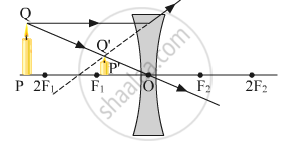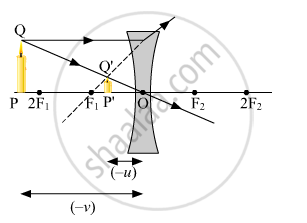# Draw a Ray Diagram to Show the Formation of Image by a Concave Lens When an Object is Placed in Front of It. - Science

(a) Draw a ray diagram to show the formation of image by a concave lens when an object is placed in front of it.

(b) In the above diagram mark the object distance (u) and the image distance (v) with their proper signs (+ve or –ve as per the new Cartesian sign convention) and state how these distances are related to the focal length (f) of the concave lens in the case.

(c) Find the nature and power of a lens which forms a real and inverted image of magnification –1 at a distance of 40 cm from the optical centre.

#### Solution

(a)(b) The object distance (u) and the image distance (v) are indicated in the figure given below.Since both the image and the object lie in the direction opposite to the direction of the incoming ray, both will be negative. The relation between uv and f given by the lens formula is

1/f=1/v-1/u

As both u and v are negative, the above equation will be changed to

1/f=1/((-v))-1/((-u))

1/f=-1/v+1/u

1/f=1/u-1/v

We know that the focal length of a concave lens is negative. Using the same argument, the above equation will be changed to

1/-f=1/u-1/v

1/f=1/v-1/u

(c) Given:-

u = −40 cm

m = −1

Since magnification is given as

m=v/u

rArrv="mu "=(-1)xx(-40)=40" cm"

Focal length can be calculated as

1/f=1/v-1/u=1/40-1/((-40))=1/20

rArrf=20" cm"

Now, the power of the convex lens can be calculated as

P=1/f(m)=100/20="5 D"

Since the power of the lens is positive, the lens will be converging in nature.

Concept: Convex Lens
Is there an error in this question or solution?
2015-2016 (March) Delhi Set 2

Share